# Internet Problem Solving Contest

## Solution to Problem B – Bishopian paths

Let’s assume that r ≤ c. (Otherwise we solve the problem for swapped dimensions and transpose its solution.)

If r = 1 then bishop cannot move along the diagonals anywhere and thus the path can only consist of a single square. This is possible for the instance with c = 2 for white squares and 2 ≤ c ≤ 3 for black squares.

All instances with r = 2 can be solved by a zig-zag pattern: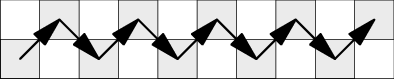The solutions above work both in B1 and B2. Below we consider each subproblem separately for larger chessboards.

### Easy subproblem B1

The ability to move the bishop along diagonals to any square enables a bishopian path in most instances.

For r = 3 one solution for each of the smallest few instances is shown on the following pictures. It is easy to generalize these patterns to chessboards with more columns.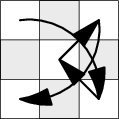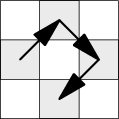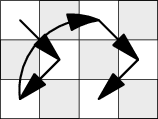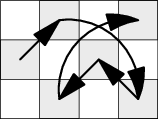There are some general patterns that essentially work for all larger chessboard dimensions, only with slight variations due to the parity of the dimensions and the chosen color. Few examples of such general patterns are shown below.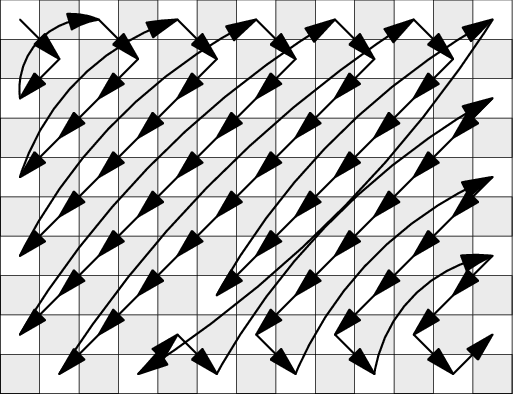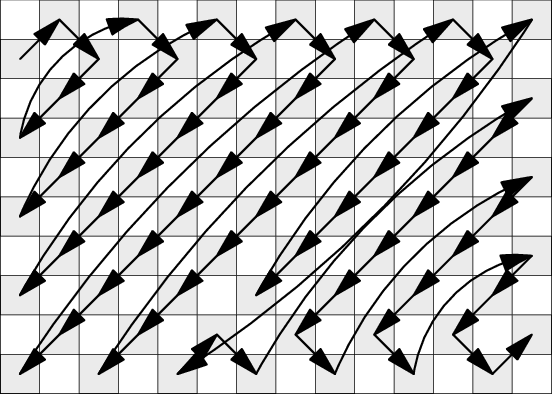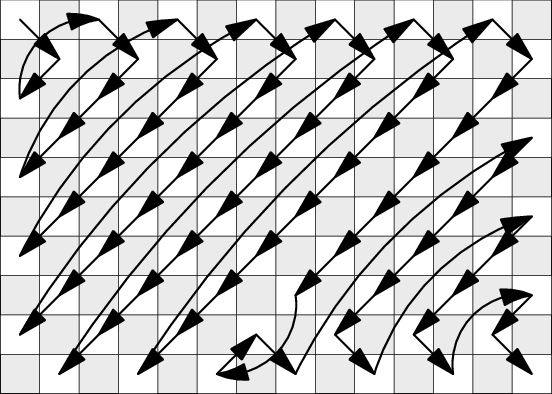### Hard subproblem B2

Once we introduce additional constraints on the bishopian path the problem changes substantially. Perhaps counterintuitively, most of the instances are impossible to solve.

First of all, let’s realize that a non-intersecting bishopian path (“NI bishopian path” below) has another equivalent definition: it is a bishopian path in which the bishop always moves by a single square only. (A path that is non-intersecting cannot contain longer jumps. Each of the cells that has been jumped over has to be visited at some other point, and doing so will create an intersection. On the other hand, any bishopian path that only contains moves of length 1 is clearly a non-intersecting path.)

Theorem 1. If 3 ≤ r ≤ c, then no NI bishopian path on white squares exists.

Proof: In addition to the square (1, 1) at least one other corner square of the chessboard is white. (Its location is determined by the parity of r and c.) Each of these corner squares is reachable only from a single white square and such squares thus have to be the endpoints of every NI bishopian path.

If r = 3, then the squares (1, 1) and (3, 1) each have only one neighbor, so they have to be the beginning and the end of the path. However, they both have the same neighbor: square (2, 2). This forces a path that consists of just these three cells and cannot be extended to other columns.

For r > 3, WLOG we can assume that the NI bishopian path will start in the top-left square (1, 1). Then it necessarily has to continue to (2, 2) from where we have three possibilities: (1, 3), (3, 3) and (3, 1). Whichever square we pick, there will remain at least one white square which is now reachable from a single white square. (In the figure below it is either square A or B.)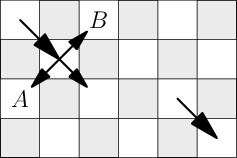Hence we now have at least three white squares which are each reachable from a single white square: the starting corner (1, 1), the other white corner, and one of A or B. This concludes our proof: a path cannot have three endpoints and thus the NI bishopian path cannot exist.

Theorem 2. There is no NI bishopian path on black squares if at least one of r and c is even.

Proof: It is easy to see that there are two black corners. We can swap the colors and rotate the chessboard so that the top left square is white. Theorem 1 now applies.

What remains to solve are the instances where both r and c odd, and we want a path on black squares.

Theorem 3. For any NI bishopian path on black squares with r and c odd: at least one endpoint of the path is located on the border of the chessboard. (I.e., there is an endpoint (x, y) such that x ∈ {1, r} or y ∈ {1, c}.)

Proof: By contradiction. Let’s assume that we have a NI bishopian path with no endpoint on the border The path has to enter and leave each square on the border of the chessboard at some point. Drawing these parts of the path results in a single closed path, as depicted on the following figure.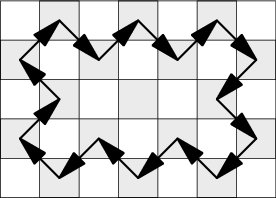All edges on this cyclic path are forced, and therefore there cannot be any NI bishopian path – those don’t contain cycles.

Theorem 4. For any instance on black squares with r and c odd, there exists a NI bishopian path in the r × c instance if and only if there exists a NI bishopian path in the instance (r + 4)×(c + 4).

Proof: We will first prove the direction from r × c to (r + 4)×(c + 4). From Theorem 3 we know that at least one of the endpoints of a NI bishopian path for the r × c board must be located on the border. This path can then be extended by a zig-zag pattern (as can be seen on figures below) to a NI bishopian path on the (r + 4)×(c + 4) chessboard.

Now let’s assume we have found a NI bishopian path on chessboard (r + 4)×(c + 4). We will discuss its properties which will enable us to shorten it to the instance r × c.

Let S1 be the set of black squares at the border of the chessboard. Let S2 be the set of black squares that would be at the border if we removed the set S1.

The set S1 contains either one or both ends of the path. Except for these one or two squares, each other square of S1 is an inner square of the path, which means that it’s connected to both of its neighbors (squares in S2). This induces a zig-zag pattern between squares of S1 and S2 as shown in the figure below.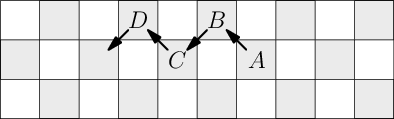The pattern will be formed around the entire chessboard, except for the one or two places where the path has an endpoint. The figures below show the main alternatives: one vs. two endpoints which may appear in a corner or on a side. (The case where one endpoint is in a corner and one is on a side may also occur but is omitted from these figures.)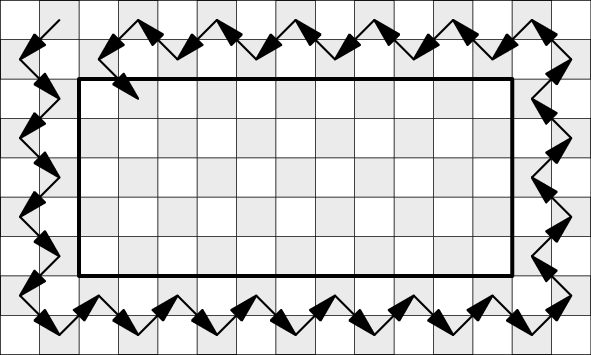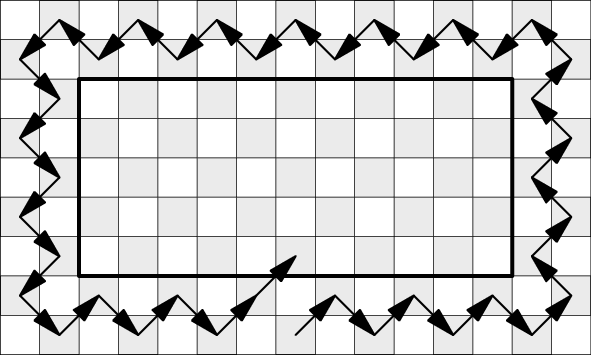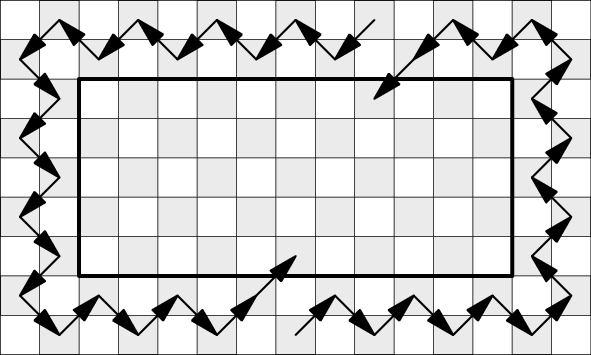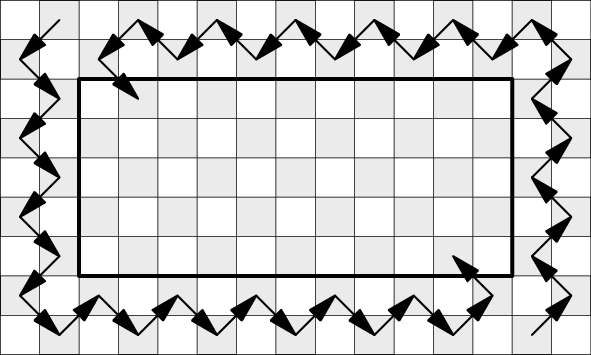In all the cases the zig-zag pattern will eventually move from S1 and S2 to inner part of the chessboard with dimensions r × c. As we can easily see, the remaining part of the original NI bishopian path will always be a NI bishopian path on the inner r × c part of the chessboard, which is precisely what we wanted to prove.

Theorem 5. For any instance on black squares with r and c odd, a solution exists if and only if |r − c|≤2.

Proof: We can use Theorem 4 to repeatedly shrink the rectangle until one of its dimensions becomes 1 or 3. The only rectangles with these dimensions that have a valid solution are shown in the figures below. (As a notable slightly-special case, when shrinking a 5 × 5 instance to a 1 × 1 instance, the entire path on the 5 × 5 instance lies on the boundary, it never enters the middle 1 × 1 area, as there are no black squares there. Feel free to check that a 5 × 5 instance has a valid solution.)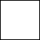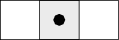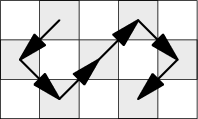Acknowledgement. Parts of the proofs shown above were adapted from a paper by G. R. Sanchis and N. Hundley.